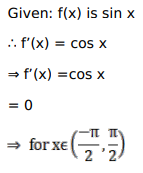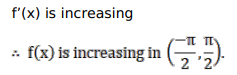# Solve this following

Question:

Mark $(\sqrt{)})$ against the correct answer in the following:

$f(x)=\sin x$ is increasing in

A. $\left(\frac{\pi}{2}, \pi\right)$

B. $\left(\pi, \frac{3 \pi}{2}\right)$

C. $(0, \pi)$

D. $\left(\frac{-\pi}{2}, \frac{\pi}{2}\right)$

Solution: